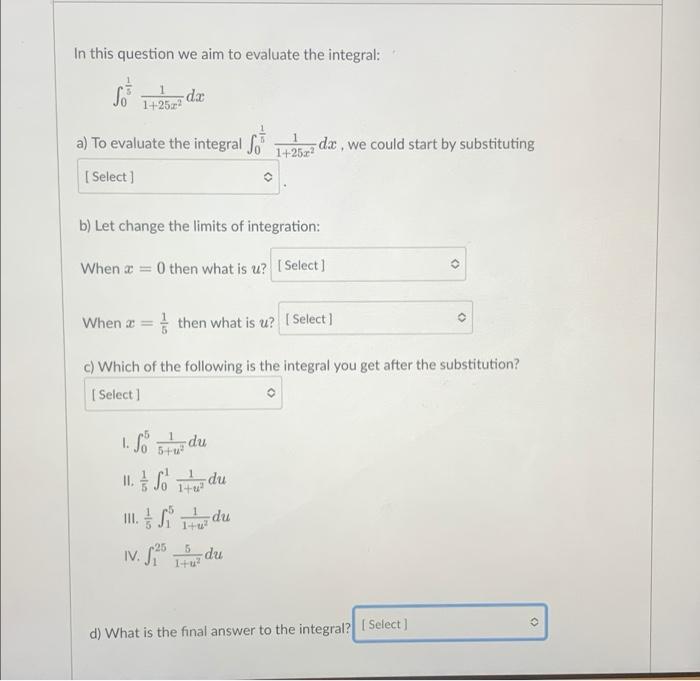# Question Solved1 Answerdx In this question we aim to evaluate the integral: So +257 da 14252 a) To evaluate the integral So 142622 dx , we could start by substituting Select) b) Let change the limits of integration: When x = 0 then what is u? [ Select] When a = ☆ then what is u? | Select] c) Which of the following is the integral you get after the substitution? [Select 1 5+ • 11, A 1. So btu du 1 g So itu du II. S Tundu IV. $25 e du . 1+ 5 Itu? ( d) What is the final answer to the integral? 1 Select)KQ60GC The Asker · CalculusTranscribed Image Text: dx In this question we aim to evaluate the integral: So +257 da 14252 a) To evaluate the integral So 142622 dx , we could start by substituting Select) b) Let change the limits of integration: When x = 0 then what is u? [ Select] When a = ☆ then what is u? | Select] c) Which of the following is the integral you get after the substitution? [Select 1 5+ • 11, A 1. So btu du 1 g So itu du II. S Tundu IV.$25 e du . 1+ 5 Itu? ( d) What is the final answer to the integral? 1 Select)
More
Transcribed Image Text: dx In this question we aim to evaluate the integral: So +257 da 14252 a) To evaluate the integral So 142622 dx , we could start by substituting Select) b) Let change the limits of integration: When x = 0 then what is u? [ Select] When a = ☆ then what is u? | Select] c) Which of the following is the integral you get after the substitution? [Select 1 5+ • 11, A 1. So btu du 1 g So itu du II. S Tundu IV. \$25 e du . 1+ 5 Itu? ( d) What is the final answer to the integral? 1 Select)